# Small Simplicity

## Measure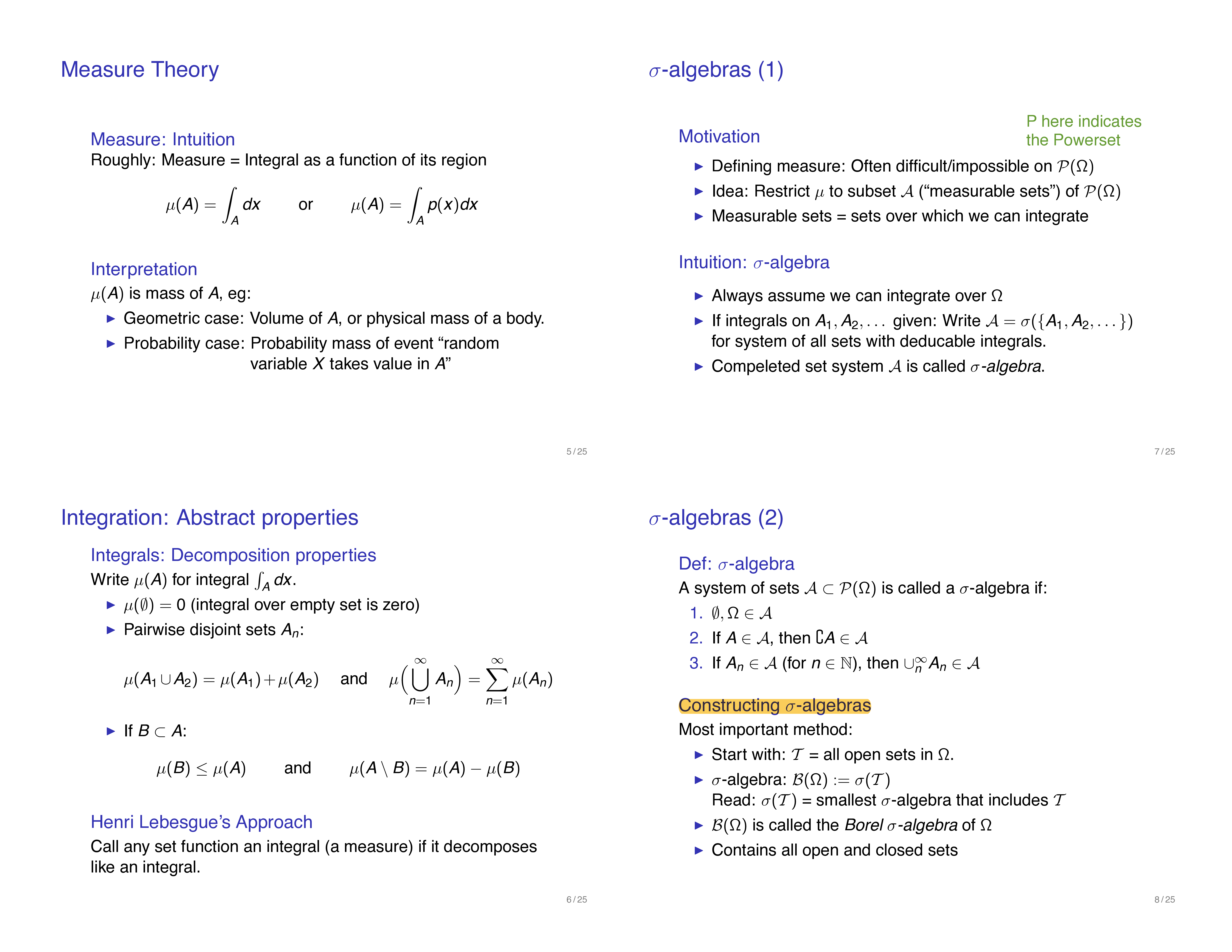- Intuition: roughly a measure is an integral as a function of its region

$$\mu(A) = \int_{A} dx ~~\text{or,} ~~~\mu(A) = \int_{A} p(x) dx$$

For example, in geometric case, $$\mu(A)$$ can be interpreted as a (physical) length (if $$A$$ is one dimensional), mass (if $$A$$ is two dimensional), or volumn (if three-dim) of a region $$A$$. In the case that $$\mu$$ is a measure of probability, $$\mu(A)$$ is the probability mass of event, "random variable $$X$$ takes values in the set $$A$$ (also called event $$A$$)"

## Density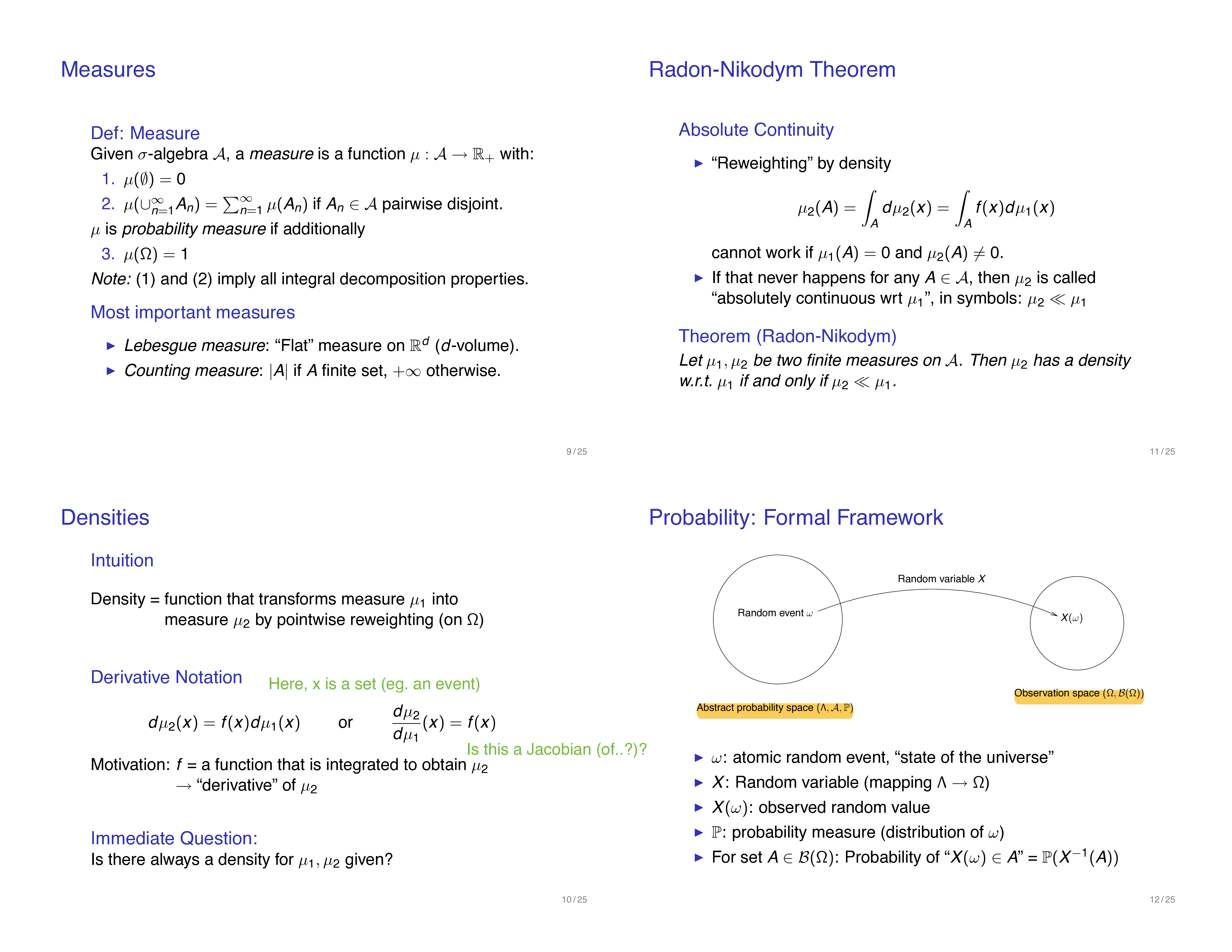A (probability) density is a function that transforms one measure to another measure by pointwise reweighting (on the abstract sample space $$\Omega$$)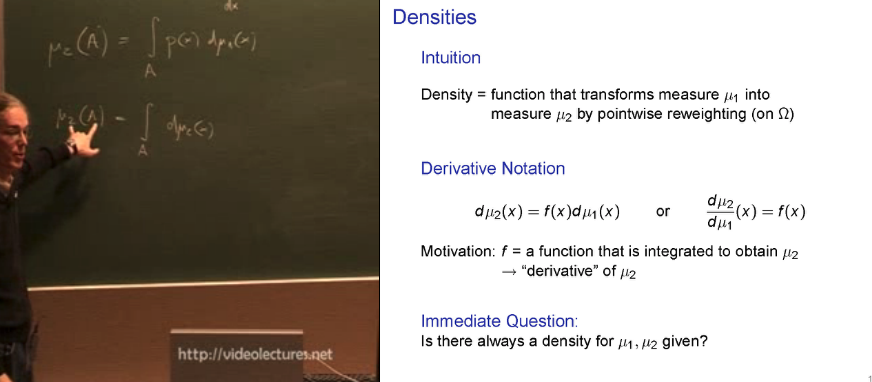## Measure-theoretic formalism for Probability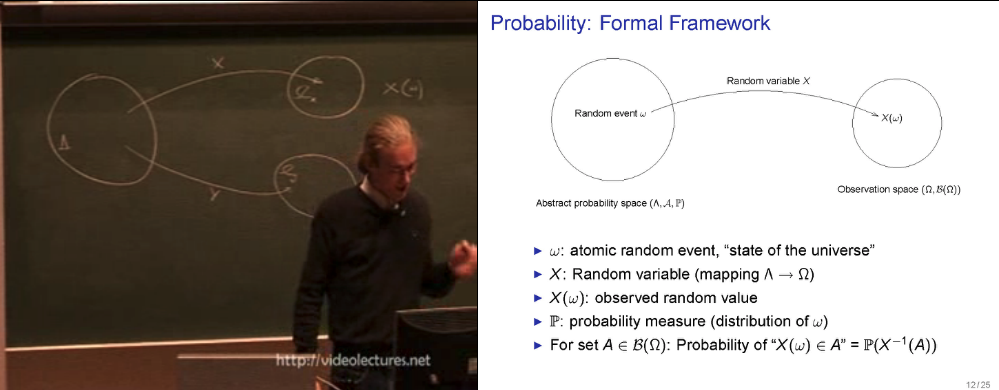• abstract probability space vs. observation space Think of the abstract probability space as the entire system of the universe. A point in the space is a state of the universe (eg. a long vector of values assigned to all existing atoms' states). We often don't have a direct access to this "state", ie. it is not fully observable to us. Instead we observe/measure variables that are some functions of this atomic configuration/state ($$w$$). This mapping from a state of the universe to a value that the variable of our interest is observed/measured to take is called a "Random Variable".

## Random Variable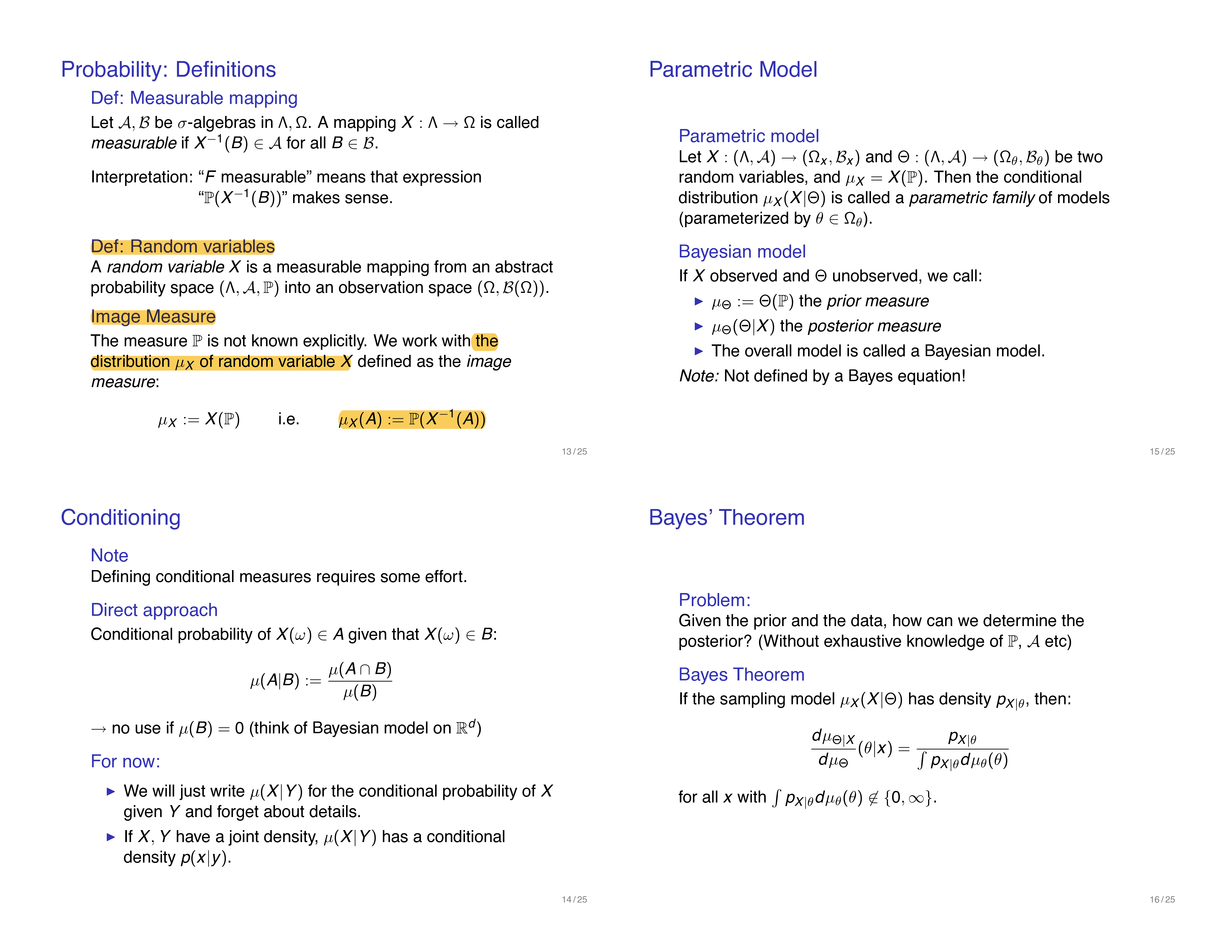- is a function that maps a outcome in the abstract probability space's sample space $$\Lambda$$ to the sample space of the observation space $$\Omega$$ (often $$\mathbb{R}$$) - it is the key component that connects the abstract probability space (which we don't get to directly observe) to the observation space - Image measure $$\mu_{X}$$ is the (derived/induced) measure on the observation space that is related to the abstract probability space via the random variable $$X$$. - We need it since the measure on the abstract probability space $$\mathbb{P}$$ is not known explicitly, but we need to have a way to descirbe the measure of sets in the Borel set of the observation space. - To assign measures to an event in the observation space, we use "Image measure" $$\mu_{X}$$ which is linked to $$\mathbb{P}$$ via:

$$\mu_{X}(A) := \mathbb{P}(X^{-1}(A))$$

- In other words, we compute the probability measure of an event $$A$$ (ie.the probability that the random variable X takes a value in the set A) by: 1. Map the set A in the observation space to a space in the abstract probability space, $$A^{\leftarrow} = X^{-1}(A)$$ 2. Compute the probability of event $$A^{\leftarrow}$$ using $$\mathbb{P}$$

## Source

• Theoretical Foundations of Nonparametric Bayesian Models, by P.Orbanz. MLSS2009: video part 1, 2. Slides 1, 2 Great introduction of measure theory just as much in detail to be relevant for statistics (and nonparametric Bayesian models)

## More resources

• MLSS09 all lecture and slide links: here

## Resource

• Lec on VAE, by Ali Ghodsi: This lecture motivates KL Divergence as the measurement of difference in the average information content of two random varialbes, whose distributions are $$p$$ and $$q$$ in in the article.
• Wiki: It clears up different terminologies that are (misused) to refer to the KL.
• Information Theory for Intelligent People, by Simon Dedeo
• It gives a great example of "answering 20 questions" problem as a way to think about basic concepts in info theory, including entropy, KL divergence and mutual information.
• $$H(X)$$ is equal to the average length of an arbitrary tree, which is the number of questions to get to choice $$x$$
• "(Using $$H(X)$$,) (f)or any probability distribution, we can now talk about "how uncertain we are about the outcome", "how much information is in the process", or "how much entropy the process has", and even measure it, in bits" (p.3)

## Basics of make for Reproducible Research¶

• A research project ca be seen as a tree of dependencies

• the report depends on the figures and tables
• the figurese and tables depend on the data and the analysis scripts used to process this data
• Make is a tool for creating output files from their dependencies through pre-specified rules

• Make is a build automatio tool
• Makefile: a configuratio file that contains the rules for what to build
• Make builds targets using recipes
• targets can optionally have _prerequisites
• prerequisites can be files on your computer or other targets: prerequisites == dependent files/targets
• Make figures out what to build based on the DAC of the targets and prerequisites (ie. its dependencies)
• the targets are updated only when needed, based on the modification time of their dependencies
• Phony targets (eg. all, clean): targets that don't actually create an output file
• they are always run if they come up in a dependency
• but will no longer be run if a directory/file is ever created that is called all or clean
• To define targets as Phony target, add a line at the top of the Makefile like:
.PHONY: all clean test

• Automatic Variables and Pattern Rules
• $<: first prerequisite • $@: target
• %: wildcard for pattern rules
• Variables and Functions: both use $(...) syntax. For example, ALL_CSV =$(wildcard data/*.csv)
INPUT_CSV = $(wildcard data/input_file_*.csv) DATA =$(filter-out $(INPUT_CSV),$(ALL_CSV)

## Caveats
• Use space as the delimiter. Don't use ,.
• Indent with tabs in Makefiles. Makefiles do not accept indentation with spaces
• Make executes each line of a Makefile independently in a separate subshell
• Make executes the first target when no explicit target is given

## Recommendation¶

• Put all as the first target in the Makefile
• Name the main target all: it's a convention many people follow
• all == reference to the main target of the Makefile
• In other words, this is the target that generates the main desired output(s)
• Put multiple outputs as the prerequisite of the main target (all)
• This allows the user to call make in the commandline, and get the desired output(s)
• All the other rules are there to help build that output (in the simplest case)
• Design your project's directory structure and Makefile hand in hand
• Use all capitals for variable names; define variables at the top of the Makefile
• start small and start early!

## More¶

• [SnakeMake]: a Python3-based alternative to Make

# Data Analysis with Latent Variable Models - Blei, 2014¶

Q: Why am I reading this?
A: To understnad the motivation behind latent variable models In particular, I want to be clear about the relations among: Probablistic Graphical Model vs. Latent Variable Model vs. Bayesian Inference. They all come up in a very similar setting, but what exactly are they?

Q: What is the product/outcome of this reading?

1. Be able to articulate the definition of Latent Variable Model

A latent variable model is a probabilistic model that encodes hidden patterns in the data (p.203)

2. Give an example in Text domain and Image domain where the model is used

3. Describe the general workflow using the model with Bayesian inference

(1) Build a model
Encode our assumptions about our data and hidden quantities that would have? could have? generated the observations as a joint distribution of hidden random variables and observation(aka. data) random variables.

• Output of this "build" step:
• definition of H (hidden random vars), V (observation random var)
• Description of the model in one of the specification
• Describe the model with its generative process, or
• Descrive the model with its the joint distribution ,P(H,V), or
• Describe the model with its graphical model representation

(2) Compute
Given observation data D, compute the conditional distribution of hidden variables given D (ie. the random variable here can be written as "h|x=D".

• NB: this is not the same random variable as "h".
• This computing process is often referred to as "inference" (I think?), as in "Bayesian inferece". However, this is different from the "inference" used to describe the processing of using a trained model as the test time to "infer", for example, the class of a new test image. Here, we are "inferring" the conditional random varialble h|x=Data, which is equivalent to say "compute the conditional distribution of the random variable, h|x=D.
• NB: This conditional distribution is often referred to as posterior. The reason it makes sense to call it so is because we are looking at the hidden quantities "posterior" to the data observation process. This is the term from the Bayseian community. But since our model is neither about being bayesian or not (see Dustin's post: "model is just a joint distribution of hidden and observation random variable. To compute the conditional distribution of hidden|observed data, or the predictive distribution P(Xnew|X=data), we can use either frequentist's tools (Eg. EM) or bayesian's tool(eg. hierachical something <- I don't know what this it), I will stick to calling this probability distribution as the "conditional" (as opposed to "posterior") distribution.
• so, the outputs of the "compute" step are:
• the conditional distribution, P(H|X=D)
• the predictive distribution, which can be computed from the conditional distribution above

(3) Critique [todo]

## Prelim¶

### Q1: What is a probabilisitc graphical model?¶

Stanford's CS228:

"Probabilistic graphical models are a powerful framework for representing complex domains usinb probability distributions, with numerous applications in achine learning, computer vision, natural language processing and computational biology. Graphical models bring together graphy theory and probability theory, and provide a flexible framework for modeling large collections of random variables with complex interactions"

### Q2: What is a Latent Variable Model?¶

Cross Validated:

"...latent variable models are graphical models where some variables are not seen, but are the causes to the observations. Some of the earliest models were factor analysis. Here the idea is to find a representation of data which reveals some inherent structure of the data..." - link

### Q3: What do you mean when you use "bayesisan" to describe a model or an inference method?¶

Dustin Tran's comment on calling a model "bayesian model" (See Bullet 1)

I strongely believe models should simply be gramed as a joint distribution p(x, z) for observation/data variable x and latent variables z

NB: this is in line with Blei's definition on a model, provided in this article (pg. 207)

A model is a joint distribution of x and h, p(h,x | hyperparam) = p(h|hyperparam) * p(x|h), which formally describes how the hidden variables and observations interact in a probability distribution

Dustin continues his comment on calling a model either "bayesian" or "frequentist". He argues this is not the right way to communicate because "there is no difference!"

... They are all just "probabilistic models". The statistical methodology -- whether it be Bayesian, frequentist, fiducial, whatever -- is about how to reason with the model given data. That is, they are about "inference" and not the "model" (Bullet 2)

This comment really clarifies my confusion on when to use the adjective "Bayesian" (ie. to describe a model? or a method of inference?):

• "Bayesian" approach is one way to do your inference (eg. compute the conditional distribution of P(hidden vars | x = observed_data).
• NB: I'm intentionally using the term conditional distribution (rather than posterior distribution of hidden variables because "posterior" is the term most often assosiated with the Bayesian inference. But, as Dustin says, we can do inference (ie. compute -- exactly or approximately -- the conditional distribution of the random variable, (z|x=Data) using either of what we ascribe as a Bayesian tool (eg. hierachical models <-- I don't know what this is) or a frequential tool (eg. EM) .
• NB2: in Bayesian framework, "posterior" means "after observations are gathered and incorporated into our reasoning about the hidden variables, ie. those that remain unobservable"

## Sec2: Model¶

The descritpion of specifying how the observations arise from the hidden quantities (aka. the generative process of the data) is where everything starts. The story you are constructing/assuming about this generative process can be expressed in 1) plain english, 2) the joint distribution between the hidden variable and observation variables, and how the joint distribution can be factored, and 3) the probabilistic graphical model. So the first thing is to write this generative process (btw this is "your" choice, "your" story, ie, the assumptions you choose/hypothesize).

• Step 1: Write in plain english what your hidden quantities that are assumed to have given rise to the observations
• Step 2: Write in plain english how they give rise to the observations. That is, what is the dependency like between the hidden quantities and the observations?
• Step 3: Now, translate the english description (that is, the definition of your model and the dependency relations) to mathematical expression using the joint distribution (<- encodes your assumptions about the data generative process) and its facotirazation (<- encodes depedency relations)
• Step 4: Represent the joint distribution (or the generative process) as a graphical model

• Generative process

• indicates a pattern of dependence among the random variable
1. Joint distribution
2. The traditional way of representing a model is with the factored joint distribution of its hidden and observed variables
3. This factorization comes directly from the generative process
4. Graphical model

## Aside: Why a joint distribution?¶

Q: What can we do with a joint distribution of the hidden and observable variables? A: Joint distribution is like the root of all other distributions. For example, we can derive conditional distribution of hidden variables given observable variables taking specific values (ie. the observations in our data): P(H|X=Data).

## NB2: Why the conditional distribution of H|X=Data?¶

NB: aka. the posterior distribution of the hidden variables given observations

We use the posterior to examine the particular hidden structure that is manifest in the observed data. We also use the posterior (over the global variables) to form the posterior predictive distribution of the future data. ... In section 5, we discuss how the predictive distribution is important for checking and criticizing latent variable models
[Blei 2014, pg. 209]

## Story so far,¶

• model = joint distribution of hidden and observable variables
• Once we have a defined model, and observations (D) , then we can compute the conditional distribution of H|X=D

• what does this posterior distribution tell us?: it helps examine the partidular hidden structure that is manifest in the observed data

• From the conditional distribution, we can compute the predictive distribution of the future data (given the model and data D). This predictive distribution is important for checking and criticizing latent variable models

## Sec3: Example models¶

Q: Why am I reading this? A: What are the outcome/product of reading this section?

• Articulate the 5 example models in three ways of specficying a model. See Sec.2.1, 2.2, 2.3 for each of the three ways.
• Describe each model by its generative process (sec 2.1)
• Describe each model by its joint distribution (sec 2.2)
• Describe each model by its graphical model (sec 2.3)

Most important figure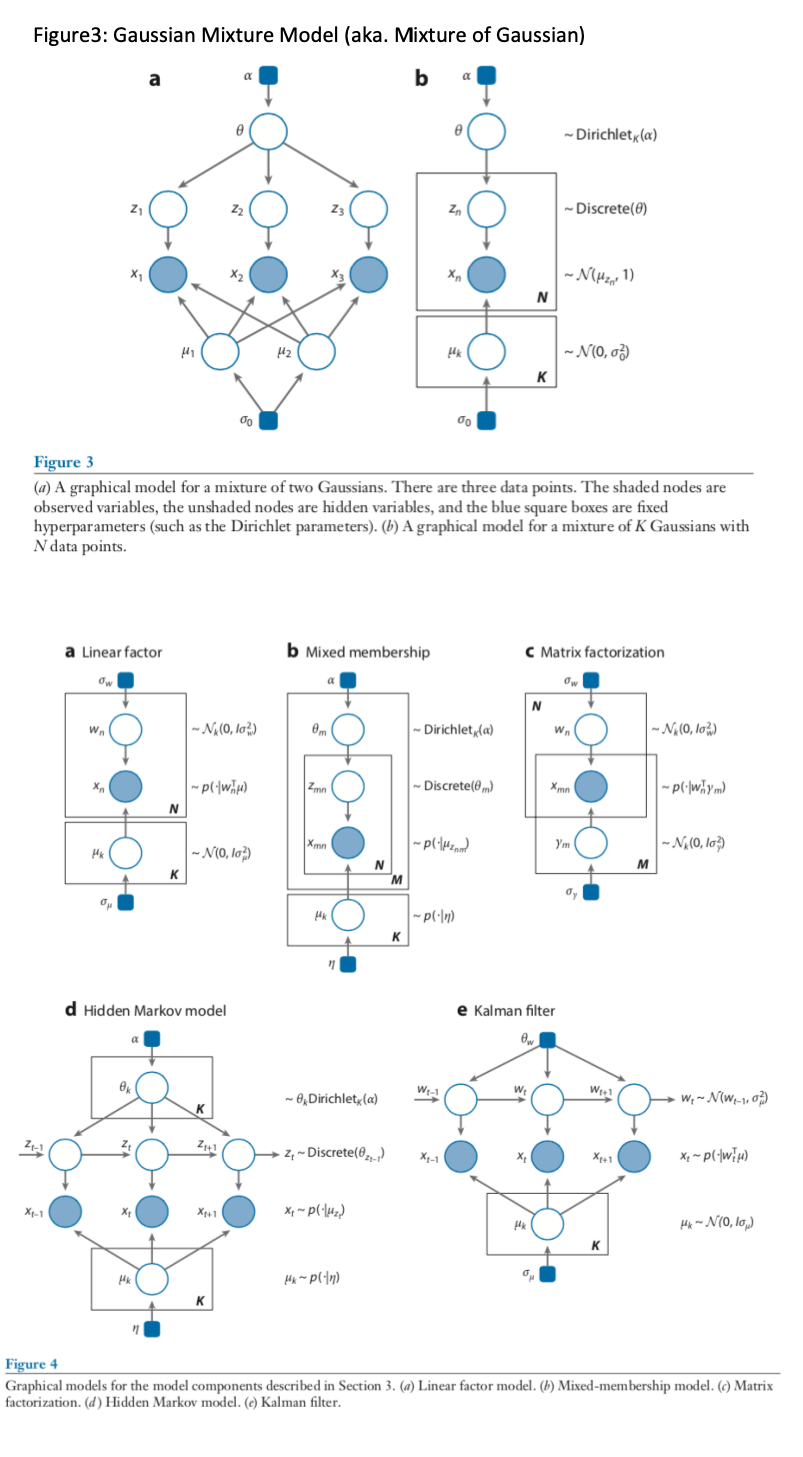[todo]

### Linear Factor model¶

• More general category is called "Factor models"
• Factor models are important as they are components in more complicated models (eg. the Kalman filter)
• Examples of statistical models that fall into this category: principal component analysis, factor analysis, canonical correlation anlysis
• Relation to Gaussian Mixture model: in Gussian mixture model, our z_n's are discrete random vars. Factor model's use 'continuous` hidden variable z.

• Generative process

• Joint Distribution
• Represent the model as a graphical model

### Kalman filter model¶

Running list of definitions Running list of terms I can't give a definition or construct an example/story out of it yet 1. A numbered 2. list * With some * Sub bullets
Next → Page 1 of 5